8th Grade Math Home > Teacher Resources > Activities > Exploring Linear Equations: Teacher Notes

 Exploring Linear Equations: Teacher Notes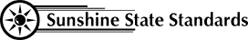MA.912.A.3.12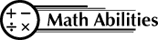Conceptual Understanding Linear Equations Graphing Procedures Procedural Knowledge Graphing Procedures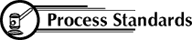Problem Solving Reasoning Connections Representation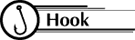When students use graphing calculators to graph equations, they are making visual models of algebraic expressions and they can readily see that an equation represents something. Graphing calculators let students create graphs quickly, too, so there is more time to ask, "What if …" or "What happens if I do this …?"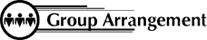Students work individually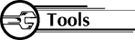Graphing Calculators Overhead CalculatorAdjust the window on the calculator to 10 by 10. Using the overhead graphing calculator, enter the equation y = 2x + 6. Hit the graph button. Ask the class to look at the slope of the line and where it crosses the y-axis. Graph y = 2x - 6. (Leave y = 2x + 6 on screen) Ask for the similarities and differences in the two graphs. Graph y = -2x + 6. Discuss the graph of this line compared with the first two lines. Ask the class what we need to put into the calculator to create a graph that is parallel to the last line, y = -2x + 6? Using the overhead graphing calculator, enter the following equations and graph: y = .5x + 7 and y = .5x - 7 Discuss what is the same about the two equations and what is different. Discuss what makes these two lines different from the first set of lines. Ask what two equations they need to put into the calculator to get intersecting parallel lines with the same y intercept. Let the class practice and raise their hands when they get it. y = -.5x + 7 and y = -.5x – 7 Now I am going to put a graph on the calculator and I want you, by observing the slope and y intercept, to guess what four equations I used to make the graph. y = -3x +4 y = -3x - 4 y = 3x + 4 y = 3x - 4 Add to the graph: y = 3x - 8 y = 3x + 8 y = -3x + 8 y = -3x - 8 Let the students investigate different graphs on the calculator. Pick a student to show their graphs, then let the student present the graphs on the overhead calculator and let the class, by observing the slope and y intercept, write the equations on their calculator.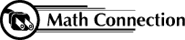As a result of this activity, students will be able to have a better understanding of the slope of a line, the y intercept, and parallel lines.

 8th Grade Math Home > Teacher Resources > Activities > Exploring Linear Equations: Teacher Notes